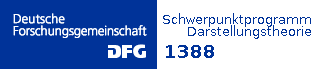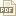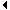﻿ DFG-Schwerpunktsprogramm Darstellungstheorie 1388

Startseite
DFG- Antrag
Gäste
Projekte
Projekte (abg.)
Publikationen
Stellen
Stellenarchiv
Terminübersicht
ArchivPublikationen4
 A | B | C| D | E | F | G | H | I | J | K | L | M | N | O | P | Q | R | S | T | U | V | W | X | Y | Z

Penkov,Ivan :
• A Bott-Borel-Weil theorem for diagonal ind-groups• Parabolic and Levi subalgebras of finitary Lie algebras• Parabolic Subgroups of Real Direct Limit Groups• Annihilators of highest weight sl(∞) –modules• On the Barth-Van de Ven-Tyurin-Sato theorem• A Categorification of the Boson-Fermion Correspondence via Representation Theory of sl(∞)• Levi Components of Parabolic Subalgebras of Finitary Lie Algebras• Schubert Decompositions for Ind-Varieties of Generalized Flags• On the structure of the Fundamental series of Generalized Harish-Chandra Modules• Linear Ind-Grassmannians• Infinite Kostant cascades and centrally generated primitive ideals of U (n) in types A(∞), C(∞)• A Koszul category of representations of finitary Lie algebras• On ideals in U(sl(∞)), U(o(∞)), U(sp(∞))• Categories of integrable sl(∞)-, o(∞)-, sp(∞)• Algebraic methods in the theory of generalized Harish-Chandra modules• Tensor Representations of Mackey Lie algebras and their dense subalgebras• Triviality of Vector Bundles on Twisted Ind-Grassmannians• On Ideals in the Enveloping Algebra of a Locally Simple Lie Algebra• Ordered tensor categories and representations of the Mackey Lie algebra of infinite matrices• Real group orbits on flag ind-varieties of SL(∞,ℂ)• On the categories of admissible (g,sl(2))-modulesPercher, Tobias :
• Multiplicity-free super vector spaces• Classification of skew multiplicity free modulesPerling, Markus :
• Examples for exceptional sequences of invertible sheaves on rational surfaces• Exceptional Sequences of Invertible Sheaves on Rational SurfacesPezzini, Guido :
• Wonderful subgroups of reductive groups and spherical systems• The spherical systems of the wonderful reductive subgroups• Primitive wonderful varieties, to appear in Math. Z.• Orbits of strongly solvable spherical subgroups on the flag variety• Automorphisms of wonderful varieties• Basics of the structure of spherical varieties• Spherical subgroups of Kac-Moody groups and transitive actions on spherical varieties• On reductive automorphism groups of regular embeddings• Combinatorial characterization of the weight monoids of smooth affine spherical varieties• On the W-action on B-sheets in positive characteristic

Reineke, Markus :
• Cohomology of quiver moduli, functional equations, and integrality of Donaldson-Thomas type invariantsRöhrle, Gerhard :

• On 1-dimensional representations of finite W-algebras associated to simple Lie algebras of exceptional type• Closed Orbits and uniform S-instability in Geometric Invariant Theory• Complete Reducibility and Conjugacy classes of tuples in Algebraic Groups and Lie algebras• Complete reducibility and separable field extensions• The strong Centre Conjecture: an invariant theory approach• Invariants of reflection groups, arrangements, and normality of decomposition classes in Lie algebras• $G$-complete reducibility and semisimple modules• Complete reducibility and Steinberg endomorphisms• On the irreducibility of symmetrizations of cross-characteristic representations of finite classical groups• On commuting varieties of nilradicals of Borel subalgebras of reductive Lie algebras• Equivariant K-theory of generalized Steinberg varieties• G-complete reducibility in non-connected groups• Spherical subgroups in simple algebraic groupsSambale, Benjamin :
• On the Brauer-Feit bound for abelian defect groups• 2-blocks with abelian defect groupsSchick, Thomas :
• Homotopy groups of the moduli space of metrics of positive scalar curvature• A Geometric Description of Equivariant K-Homology for Proper Actions• Smooth orbifold $K$-TheorySchweigert, Christoph :
• Twenty-five years of two-dimensional rational conformal field theory• Defect lines, dualities, and generalised orbifolds• The three-dimensional origin of the classifying algebra• A Geometric Construction for Permutation Equivariant Categories from Modular Functors• Equivariant Modular Categories via Dijkgraaf-Witten Theory• On the Brauer Groups of Symmetries of Abelian Dijkgraaf-Witten Theories• Partially dualized Hopf algebras have equivalent Yetter-Drinfel'd modules• Higher genus mapping class group invariants from factorizable Hopf algebrasSoergel, Wolfgang :
• Modular Koszul Duality• Proper base change for separated locally proper maps• Unicity of graded covers of the category O of Bernstein-Gelfand-GelfandStroppel, Catharina :
• The sl(n)-WZNW Fusion Ring: a combinatorial construction and a realisation as quotient of quantum cohomology• Highest weight categories arising from Khovanov's diagram algebra IV: the general linear supergroup• Highest weight categories arising from Khovanov's diagram algebra III: category O• Highest weight categories arising from Khovanov's diagram algebra II: Koszulity• Highest weight categories arising from Khovanov's diagram algebra I: cellularityWeidner, Jan :
• Grassmannians and Koszul dualityWibmer, Michael :
• Grassmannians and Koszul duality• Difference algebraic relations among solutions of linear differential equations• $\sigma$-Galois theory of linear difference equations• Skolem-Mahler-Lech type theorems and Picard-Vessiot theory• Difference Galois theory of linear differential equations• An intrinsic characterization of Picard-Vessiot extensions• A Chevalley theorem for difference equations• Existence of $\partial$-parameterized Picard-Vessiot extensions over fields with algebraically closed constants• On the Galois theory of strongly normal differential and difference extensions, Séminaires et Congrès 27, 221-240, 2012. (nicht online vorhanden)

Thiel, Ulrich :
• A Counter-Example to Martino’s Conjecture About Generic Calogero–Moser Families• Champ: a Cherednik algebra Magma package• Decomposition Matrices are Generically TrivialYang, Dong :
• Relative singularity categories I: Auslander resolutions• Ladders and simplicity of derived module categories•Zurück1 | 2 | 3 | 4

| Impressum |

News

• Die Vortragsfolien der Vorträge auf der Schwerpunkttagung 2015 finden sie hier.
• Conference Volume hier.